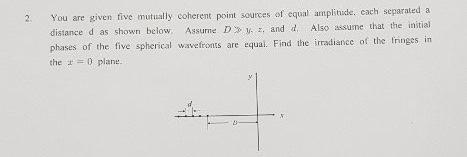Create an Account

Home / Questions / You are given five mutually coherent point sources of equal amplitude, cach separated a di...

You are given five mutually coherent point sources of equal amplitude, cach separated a distance d as shown below Assume Dy, and Also assume that the initial phases of the five spherical

You are given five mutually coherent point sources of equal amplitude, cach separated a distance d as shown below Assume Dy, and Also assume that the initial phases of the five spherical wavefronts are equal. Find the irradiance of the fringes in the = planeMay 28 2021 View more View LessSubscribe To Get Solution